# Quiz 32: The Debate Over Monetary and Fiscal Policy

Business

At a higher rate of interest, the returns yield from savings will also be high. Since the return accrued through savings is high, people prefer savings rather than cash in hand. This is because the opportunity cost of keeping cash in hand is higher than savings. Therefore, at a higher rate of interest people prefer to hold less cash in hand. As a result, the existing money stock will circulate faster in the market/with people. The faster the money circulates the velocity of money will increase. Hence, at a higher rate of interest, the velocity of money would be higher.

Definition of M1 M1 money refers to the total amount of money in the circulation and savings with banks and other saving institutions. Velocity of money Velocity of money refers to how many times the total money supply circulated in the economy in a given period of time. Calculation of velocity of money According to Fisher's quantity theory of money, the demand for money and supply of money can be written as follows: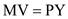……(1) Where, M = Money supply V = Velocity of money P = General price level Y = Total output MV refers to the supply of money and PY refers to the demand for money. To calculate the velocity of money, Equation (1) can be written as follows: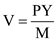……(2) In Equation (2), PY refers to gross domestic product and M refers to monetary base. Hence, Equation (2) can be rewritten as: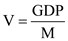……(3) Individual velocity of money in the year 2006 It is assumed that: Individual's cash in hand for the 12 months of 2006 is \$1,350. Savings with banks and other saving institutions is \$750. Hence, the total money that the individual has is \$2,100. Gross domestic product in the year 2006 was \$13,399. Substitute this information in Equation (3) to obtain the velocity of money for the individual. This can be written as follows:Hence, the velocity of money for the individual in the year 2006 was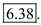United States velocity of money in the year 2006 Money supply of United States in the year 2006 was \$1,367, and the gross domestic product was \$12,399. Substitute this information in Equation (3) to obtain velocity of money for the United States. This can be written as follows:Hence, the velocity of money for the United States in the year 2006 was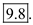Conclusion • The velocity of the money for the individual in the year 2006 was 6.38. • The velocity of the money for United States in the year 2006 was 9.8. Hence, the velocity of money in the year 2006 for the individual is different from that for the United States.

Velocity of money Velocity of money refers to the number of times the total money supply is circulated in the economy in a given period of time. Calculation of velocity of money According to Fisher's quantity theory of money, the demand for money and supply of money is written as follows:……(1) Where, M = Money supply V = Velocity of money P = General price level Y = Total output MV refers to the supply of money and PY refers to the demand for money. To calculate the velocity of money, Equation (1) can be written as follows:……(2) In Equation (2), PY refers to Gross Domestic Product and M refers to monetary base, which consists of M1 and M2. Federal Reserve and the velocity of the money Banks play an important role in the money transaction and monetary base. Banks provide a variety of services to the customers for transactions such as debit/credit cards, online payments, mobile banking, etc. Therefore, these transactions made changes in the M1 or M2 causes change in the velocity since M1 and M2 are involved in the calculation of velocity. Hence, Federal Reserve has to formulate targets for M1 or M2.